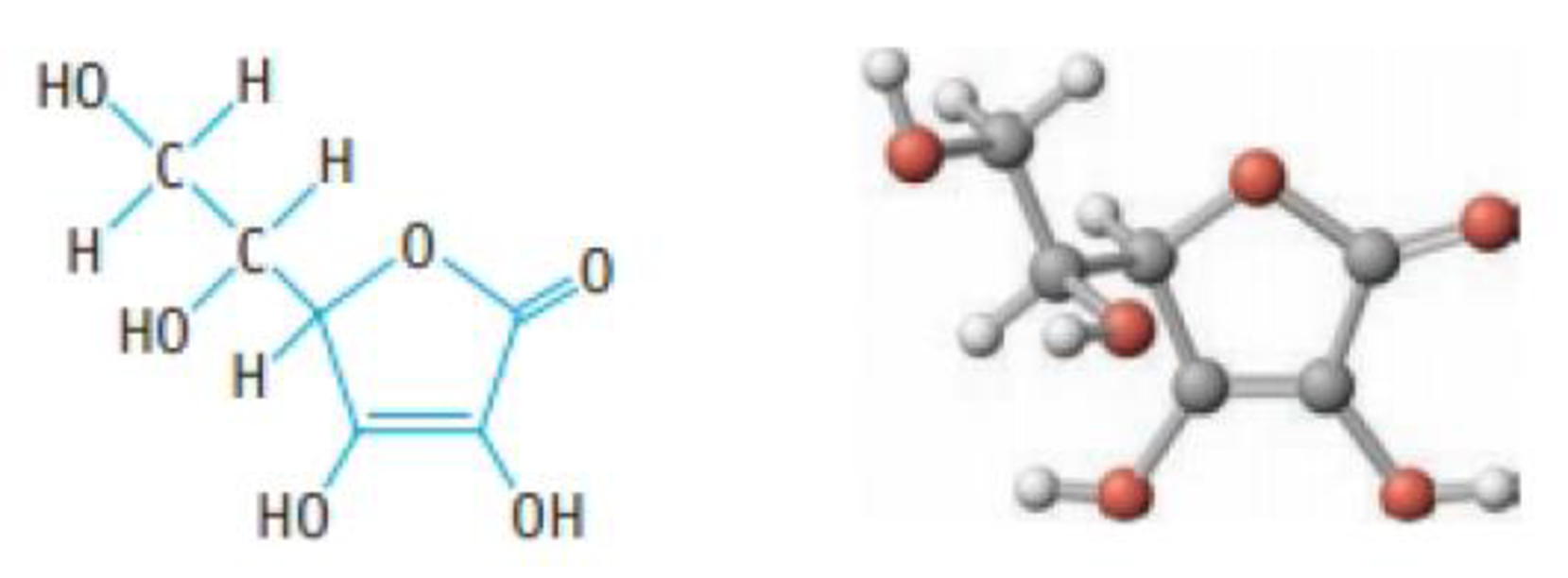# Ascorbic acid (vitamin C, C 6 H 8 O 6 ) is a diprotic acid ( K a 1 = 6.8 × 10 −5 and K a 2 = 2.7 × 10 −12 ). What is the pH of a solution that contains 5.0 mg of acid per millilitre of solution? Ascorbic acid### Chemistry & Chemical Reactivity

9th Edition
John C. Kotz + 3 others
Publisher: Cengage Learning
ISBN: 9781133949640

#### Solutions

Chapter
Section### Chemistry & Chemical Reactivity

9th Edition
John C. Kotz + 3 others
Publisher: Cengage Learning
ISBN: 9781133949640
Chapter 16, Problem 72PS
Textbook Problem
972 views

## Ascorbic acid (vitamin C, C6H8O6) is a diprotic acid (Ka1 = 6.8 × 10−5 and Ka2 = 2.7 × 10−12). What is the pH of a solution that contains 5.0 mg of acid per millilitre of solution?Ascorbic acid

Interpretation Introduction

Interpretation:

The pH of a solution has to be calculated when 5.0 mg of acid per millilitre of solution of ascorbic acid.

Concept introduction:

If a molecule donate only one hydrogen atom then the acid is called a monoprotic acid.

Example: HCl, HBr and HClO3

If each molecule can donate two or more hydrogen ions, the acid is a polyprotic acid.

A diprotic acid, such as sulfuric acid, H2SO4 has two acidic hydrogen atoms.

Some acids, such as phosphoric acid, H3PO4 are triprotic acids.

Polyprotic bases:

Can accept more than on proton (H+). So, it can react with water many times to produce many hydroxide ions (OH).

Diprotic bases:

Can accept two protons.

Example: Sulphate ion SO4(aq)2-

### Explanation of Solution

Let’s calculate the concentration of ascorbic acid

Given amount = 5.0mg

Molecular mass of ascorbic acid = 176g/mol

Molarity of ascorbic acid = Mass of ascorbic acid(g)Molar mass of ascorbic acid(g/mol)×1000Volume of solution(mL)=   [5.0mgC6H8O6×1g1000mg]176.g/molC6H8O6×(10001mL)=   2.84×10-2M

Ascorbic acid is a diprotic acid. The ionization steps of ascorbic are as follows.

First ionization:C6H8O6(aq) + H2O(aq)C6H7O6-(aq) + H3O+(aq)Equilibrium expression:Ka1 = [C6H7O6-][H3O+][C6H8O6]Ka1= 6.8×10-5Second ionization:C6H7O6-(aq) + H2O(aq)C6H6O62-(aq) + H3O+(aq)Equilibrium expression:Ka2[C6H6O62-][H3O+][C7H7O6-]Ka2= 2.7×10-12

From the Ka1 and Ka2 values,  Ka2 is smaller than the  Ka1.

Therefore, H3O+ is almost produced entirely from  Ka1.

Let’s calculate the H3O+ from  Ka1.

Enter the ICE table the concentrations before equilibrium is established, the change that occurs as the reaction proceeds to equilibrium and the concentrations when equilibrium has been achieved

### Still sussing out bartleby?

Check out a sample textbook solution.

See a sample solution

#### The Solution to Your Study Problems

Bartleby provides explanations to thousands of textbook problems written by our experts, many with advanced degrees!

Get Started

Find more solutions based on key concepts
Match the molecular model with the correct chemical formula: CH3OH, NH3, KCl, H2O.

General Chemistry - Standalone book (MindTap Course List)

According to the USDA Eating Patterns, which of the following vegetables should be limited? a. carrots b. avoca...

Nutrition: Concepts and Controversies - Standalone book (MindTap Course List)

22. Name each compound:

Chemistry In Focus

How can errors in the cell cycle lead to cancer in humans?

Human Heredity: Principles and Issues (MindTap Course List)

Why do biologists often use model organisms in their research?

Biology: The Dynamic Science (MindTap Course List)

The sperm of swim to eggs. a. mosses b. ferns c. conifers d. a and b

Biology: The Unity and Diversity of Life (MindTap Course List)

Plankton are said to be drifters, yet some of them can swim. Which is it?

Oceanography: An Invitation To Marine Science, Loose-leaf Versin

To monitor the breathing of a hospital patient, a thin bell is girded around the patient's chest. The belt is a...

Physics for Scientists and Engineers, Technology Update (No access codes included)